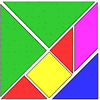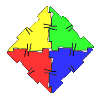#### You may also like### Tangrams

Can you make five differently sized squares from the interactive tangram pieces?### Polydron

This activity investigates how you might make squares and pentominoes from Polydron.### Construct-o-straws

Make a cube out of straws and have a go at this practical challenge.

# Counting Counters

##### Age 7 to 11Challenge Level

Jade from Coombe Girls School and Rohaan from North Cross Intermediate correctly spotted the pattern in the number of counters in each ring:

Every ring you add, you are just adding $6$ more counters than the previous ring.
Circle number excluding the counter in the middle $\times6 =$number of counters in that particular layer

Volkan and other pupils at FMV Ozel Erenkoy Isik Primary solved the second part of the problem:

At the end of the third layer, there are; $1+6+12=19$ counters.

At the end of the fourth layer, there are; $19+(3\times6)=37$ counters.

At the end of the seventh layer, there are; $37+(15\times6)=127$ counters.

At the end of the ninth layer, there are; $127+(15\times6)=217$ counters.

Some people solved this part by numbering the counters, starting from one in the middle and counting outwards. Thank you for sending in your solutions!